# Sound Intensity

Sound intensity is defined as the sound power per unit area. The usual context is the measurement of sound intensity in the air at a listener's location. The basic units are watts/m2 or watts/cm2. Many sound intensity measurements are made relative to a standard threshold of hearing intensity I0 :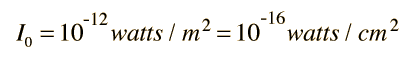The most common approach to sound intensity measurement is to use the decibel scale: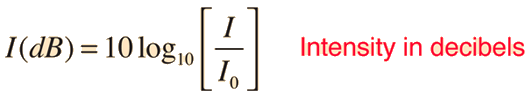Decibels measure the ratio of a given intensity I to the threshold of hearing intensity, so that this threshold takes the value 0 decibels (0 dB). To assess sound loudness, as distinct from an objective intensity measurement, the sensitivity of the ear must be factored in.

Index

Sound level measurement

Loudness concepts

 HyperPhysics***** Sound R Nave
Go Back

# Sound Pressure

Since audible sound consists of pressure waves, one of the ways to quantify the sound is to state the amount of pressure variation relative to atmospheric pressure caused by the sound. Because of the great sensitivity of human hearing, the threshold of hearing corresponds to a pressure variation less than a billionth of atmospheric pressure.

The standard threshold of hearing can be stated in terms of pressure and the sound intensity in decibels can be expressed in terms of the sound pressure: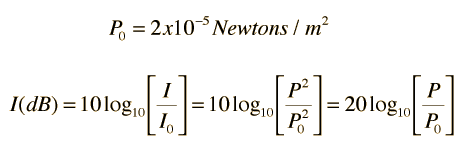The relationship of sound intensity to pressure is discussed below. The pressure P here is to be understood as the rms pressure of the collection of waves which make up the sound. The power carried by a traveling wave is proportional to the square of the amplitude. The factor of 20 comes from the fact that the logarithm of the square of a quantity is equal to 2 x the logarithm of the quantity. Since common microphones such as dynamic microphones produce a voltage which is proportional to the sound pressure, then changes in sound intensity incident on the microphone can be calculated from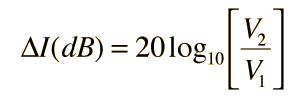where V1 and V2 are the measured voltage amplitudes .

Index

Sound level measurement

 HyperPhysics***** Sound R Nave
Go Back

# Threshold of Hearing

Sound level measurements in decibels are generally referenced to a standard threshold of hearing at 1000 Hz for the human ear which can be stated in terms of sound intensity:or in terms of sound pressure: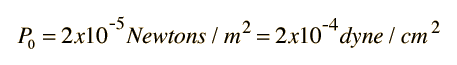This value has wide acceptance as a nominal standard threshold and corresponds to 0 decibels. It represents a pressure change of less than one billionth of standard atmospheric pressure. This is indicative of the incredible sensitivity of human hearing. The actual average threshold of hearing at 1000 Hz is more like 2.5 x 10-12 watts/m2 or about 4 decibels, but zero decibels is a convenient reference. The threshold of hearing varies with frequency, as illustrated by the measured hearing curves.

 Measured threshold as a function of frequency
Index

Sound level measurement

 HyperPhysics***** Sound R Nave
Go Back

# Threshold of Pain

The nominal dynamic range of human hearing is from the standard threshold of hearing to the threshold of pain. A nominal figure for the threshold of pain is 130 decibels, but that which may be considered painful for one may be welcomed as entertainment by others. Generally, younger persons are more tolerant of loud sounds than older persons because their protective mechanisms are more effective. This tolerance does not make them immune to the damage that loud sounds can produce.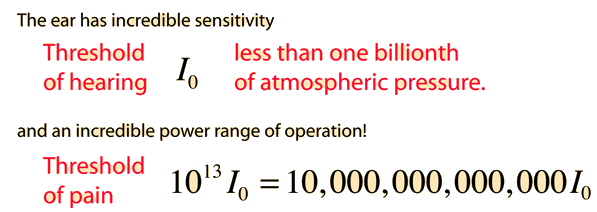Some sources quote 120 dB as the pain threshold and define the audible sound frequency range as ending at about 20,000 Hz where the threshold of hearing and the threshold of pain meet.

Index

Sound level measurement

 HyperPhysics***** Sound R Nave
Go Back

# Relation of Sound Intensity to Sound Pressure

Sound travels through air as a longitudinal wave which may contain many frequencies. The intensity of the sound may be expressed in terms of the rms pressure of the collection of waves provided that the average is over at least one period of the lowest frequency contained in the sound. The intensity relationship is analogous to the electric power relationship where the rms pressure is analogous to voltage and the wave impedance of the air is analogous to the electric resistance R.

The acoustic resistance or wave impedance R of air is calculated as the density of the air times the speed of sound in air, R = ρvs. At 20°C and atmospheric pressure this resistance is: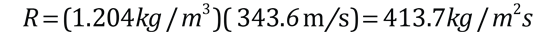Since the acoustic resistance is independent of the sound frequency, the intensity can be expressed in terms of the sound pressure level: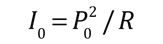The pressure P here is to be understood as the rms pressure of the collection of waves which make up the sound. Making use of the sound intensity associated with zero decibels, I0 = 10-12 watts/m2, the sound pressure level (SPL) P0 associated with the threshold of hearing isThis can be used to develop the expression for sound pressure level related to the threshold of hearing above.Index

Sound level measurement

dB SPL, Stanford

 HyperPhysics***** Sound R Nave
Go Back